Register for Maths, Science, English, Reasoning Olympiad Exams Register here | Book Free Demo for Live Olympiad Classes here| Check Olympiad Exam Dates here | Buy Practice Papers for IMO, IOM, HEO, IOEL etc here | Login here to participate in all India free mock test on every Saturday

# Chapter 4: Structure of Atom

Science

Chapter 4: Structure of Atom

Class: IX

Exemplar sheet 4

Multiple Choice Questions

Question 1

Which of the following correctly represent the electronic distribution in the Mg atom?

(a) 3, 8, 1

(b) 2, 8, 2

(c) 1, 8, 3

(d) 8, 2, 2

(b)

Question 2

Rutherford’s ‘alpha (α) particles scattering experiment’ resulted in to discovery of

(a) Electron

(b) Proton

(c) Nucleus in the atom

(d) Atomic mass

(c)

Question 3

The number of electrons in an element X is 15 and the number of neutrons is 16. Which of the following is the correct representation of the element?

(a) 3115 X

(b) 3116 X

(c) 1615 X

(d) 1516 X

(a)

Question 4

Dalton’s atomic theory successfully explained

(i) Law of conservation of mass

(ii) Law of constant composition

(iv) Law of multiple proportion

(a) (i), (ii) and (iii)

(b) (i), (iii) and (iv)

(c) (ii), (iii) and (iv)

(d) (i), (ii) and (iv)

(d)

Question 5

Which of the following statements about Rutherford’s model of atom are correct?

(i) considered the nucleus as positively charged

(ii) established that the α–particles are four times as heavy as a hydrogen atom

(iii) can be compared to solar system

(iv) was in agreement with Thomson’s model

(a) (i) and (iii)

(b) (ii) and (iii)

(c) (i) and (iv)

(d) only (i)

(a)

Question 6

Which of the following are true for an element?

(i) Atomic number = number of protons + number of electrons

(ii) Mass number = number of protons + number of neutrons

(iii) Atomic mass = number of protons = number of neutrons

(iv) Atomic number = number of protons = number of electrons

(a) (i) and (ii)

(b) (i) and (iii)

(c) (ii) and (iii)

(d) (ii) and (iv)

(d)

Question 7

In the Thomson’s model of atom, which of the following statments are correct?

(i) the mass of the atom is assumed to be uniformaly distributed over the atom

(ii) the positive charge is assumed to be uniformaly distributed over the atom

(iii) the electrons are uniformaly distributed in the positively charged sphere

(iv) the electrons attract each other to stabilise the atom

(a) (i), (ii) and (iii)

(b) (i) and (iii)

(c) (i) and (iv)

(d) (i), (iii) and (iv)

(a)

Question 8

Rutherford’s α–particle scattering experiment showed that

(i) electrons have negative charge

(ii) the mass and positive charge of the atom is concentrated in the nucleus

(iii) neutron exists in the nucleus

(iv) most of the space in atom is empty

Which of the above statements are correct?

(a) (i) and (iii)

(b) (ii) and (iv)

(c) (i) and (iv)

(d) (iii) and (iv)

(b)

Question 9

The ion of an element has 3 positive charges. Mass number of the atom is 27 and the number of neutrons is 14. What is the number of electrons in the ion?

(a) 13

(b) 10

(c) 14

(d) 16

(b)

Question 10

Identify the Mg2+ ion from the Fig.4.1 where, n and p represent the number of neutrons and protons respectively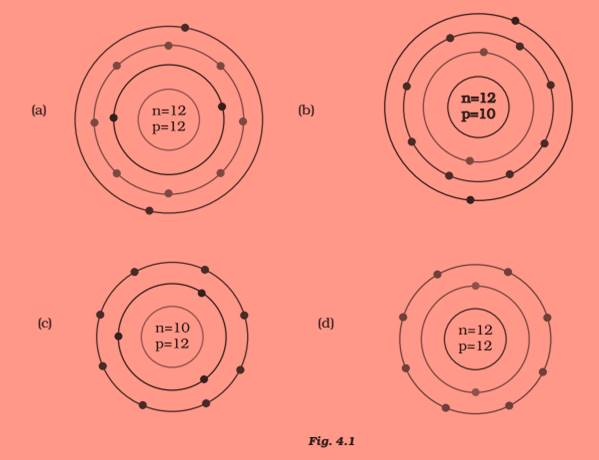(d)

Question 11

In a sample of ethyl ethanoate (CH3COOC2H5 ) the two oxygen atoms have the same number of electrons but different number of neutrons. Which of the following is the correct reason for it?

(a) One of the oxygen atoms has gained electrons

(b) One of the oxygen atoms has gained two neutrons

(c) The two oxygen atoms are isotopes

(d) The two oxygen atoms are isobars.

(c)

Question 12

Elements with valency 1 are

(a) always metals

(b) always metalloids

(c) either metals or non-metals

(d) always non-metals

(c)

Question 13

The first model of an atom was given by

(a) N. Bohr

(b) E. Goldstein

(c) Rutherford

(d) J.J. Thomson

(d)

Question 14

An atom with 3 protons and 4 neutrons will have a valency of

(a) 3

(b) 7

(c) 1

(d) 4

(c)

Question 15

The electron distribution in an aluminium atom is

(a) 2, 8, 3

(b) 2, 8, 2

(c) 8, 2, 3

(d) 2, 3, 8

(a)

Question 16

Which of the following in Fig. 4.2 do not represent Bohr’s model of an atom correctly?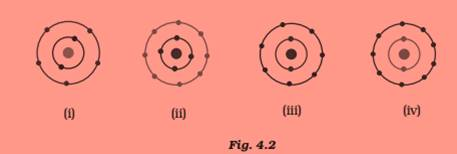(a) (i) and (ii)

(b) (ii) and (iii)

(c) (ii) and (iv)

(d) (i) and (iv)

(c)

Question 17

Which of the following statement is always correct?

(a) An atom has equal number of electrons and protons.

(b) An atom has equal number of electrons and neutrons.

(c) An atom has equal number of protons and neutrons.

(d) An atom has equal number of electrons, protons and neutrons.

(a)

Question 18

Atomic models have been improved over the years. Arrange the following

atomic models in the order of their chronological order

(i) Rutherford’s atomic model

(ii) Thomson’s atomic model

(iii) Bohr’s atomic model

(a) (i), (ii) and (iii)

(b) (ii), (iii) and (i)

(c) (ii), (i) and (iii)

(d) (iii), (ii) and (i)

(c)

Question 19

Is it possible for the atom of an element to have one electron, one proton and no neutron. If so, name the element.

Yes, it is true for hydrogen atom which is represented as 1 1H

Question 20

Write any two observations which support the fact that atoms are divisible.

Hint— Discovery of electrons and protons

Question 21

Hint— No, 35 Cl and 37 Cl are isotopes of an element.

Question 22

Why did Rutherford select a gold foil in his α–ray scattering experiment?

Hint— gold has high malleability

Question 23

Find out the valency of the atoms represented by the Fig. 4.3 (a) and (b).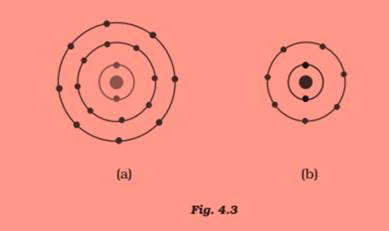(a) 0

(b) 1

Question 24

One electron is present in the outer most shell of the atom of an element X. What would be the nature and value of charge on the ion formed if this electron is removed from the outer most shell?

+ 1

Question 25

Write down the electron distribution of chlorine atom. How many electrons are there in the L shell? (Atomic number of chlorine is 17).

2, 8, 7. The L shell has eight electrons

Question 26

In the atom of an element X, 6 electrons are present in the outermost shell. If it acquires noble gas configuration by accepting requisite number of electrons, then what would be the charge on the ion so formed?

–2

Question 27

What information do you get from the Fig. 4.4 about the atomic number, mass number and valency of atoms X, Y and Z? Give your answer in a tabular form.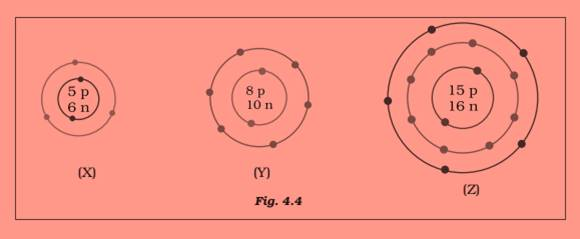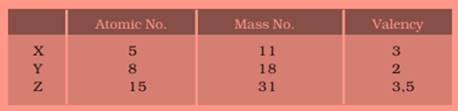Question 28

In response to a question, a student stated that in an atom, the number ofprotons is greater than the number of neutrons, which in turn is greaterthan the number of electrons. Do you agree with the statement? Justifyyour answer.

Hint— No, the statement is incorrect. In an atom the number of protons and electrons is always equal.

Question 29

Calculate the number of neutrons present in the nucleus of an element X which is represented as 3115 X

Mass number = No. of protons + No. of neutrons = 31

Number of neutrons = 31– number of protons

= 31–15 = 16

Question 30

Match the names of the Scientists given in column A with their contributions

towards the understanding of the atomic structure as given in column B

(A)  (B)

(a) Ernest Rutherford  (i) Indivisibility of atoms

(b) J.J.Thomson   (ii) Stationary orbits

(c) Dalton   (iii) Concept of nucleus

(d) Neils Bohr   (iv) Discovery of electrons

(e) James Chadwick   (v) Atomic number

(f) E. Goldstein   (vi) Neutron

(g) Mosley   (vii) Canal rays

(a) (iii)    (b) (iv)   (c) (i)   (d) (ii)

(e) (vi)  (f) (vii)   (g) (v)

Question 31

The atomic number of calcium and argon are 20 and 18 respectively, but the mass number of both these elements is 40. What is the name given to such a pair of elements?

Isobars

Question 32

Complete the Table 4.1 on the basis of information available in the symbols

given below

(a) 3517Cl   (b) 126C  (c) 8135 Br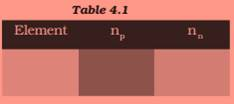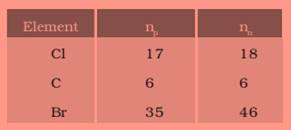Question 33

Helium atom has 2 electrons in its valence shell but its valency is not 2, Explain.

Helium atom has 2 electrons in its outermost shell and its duplet is complete. Hence the valency is zero.

Question 34

Fill in the blanks in the following statements

(a) Rutherford’s α-particle scattering experiment led to the discovery of the ———

(b) Isotopes have same ———but different———.

(c) Neon and chlorine have atomic numbers 10 and 17 respectively. Their valencies will be——

and———respectively.

(d) The electronic configuration of silicon is ———and that of sulphur is ———.

(a) atomic nucleus

(b) atomic number, mass number

(c) 0 and 1.

(d) Silicon—2, 8, 4

Sulphur— 2, 8, 6

Question 35

An element X has a mass number 4 and atomic number 2. Write the valency of this element?

Valency is zero as K shell is completely filled.

Question 36

Why do Helium, Neon and Argon have a zero valency?

Helium has two electrons in its only energy shell, while Argon and Neon have 8 electrons in their valence shells. As these have maximum number of electrons in their valence shells, they do not have any tendency to combine with other elements. Hence, they have a valency equal to zero.

Question 37

The ratio of the radii of hydrogen atom and its nucleus is ~ 105. Assuming the atom and the nucleus to be spherical, (i) what will be the ratio of their sizes? (ii) If atom is represented by planet earth ‘Re’ = 6.4 ×106 m, estimate the size of the nucleus.

(i) Volume of the sphere = (4 / 3) π r3

Let R be the radius of the atom and r be that of the nucleus.

R = 105 r

Volume of the atom = 4 /3 πR3 = 4 /3 π(105 r) 3  (given  R = 105r )

= 4/3 π rx 1015

Volume of the nucleus = 4/3 π r

Ratio of the size of atom to that of nucleus = (4/3 π rx 1015) / (4/3 π r)

= 1015

(ii) If the atom is represented by the planet earth (Re = 6.4×106m) then the radius of the  nucleus would be r= Re /105

rn = 6.4×106 / 105 m = 6.4 x 10 m

= 64 m.

Question 38

Enlist the conclusions drawn by Rutherford from his α-ray scattering experiment.

Rutherford's conclution from the α-particle scattering experiment that–

(i) Most of the space inside the atom is empty or vacant hence most of the α-particles passed through the gold foil without deflecting or deviating.

(ii) Very very few particles were deflected from their path, this indicates that all positive charges are occupies little space.

(iii) A very small fraction of α-particles were bounce of the nucleus indicates that both the positive charges and mass of the atom were concentrated in a very small portion within the atom.

Question 39

In what way is the Rutherford’s atomic model different from that of Thomson’s atomic model?

In atom model proposed by Rutherford, the electrons revolve around the nucleus in a well-defined  circular orbits. There is a positively charged centre in an atom called the nucleus and  the size of the atom  is very big as compared to the size of the nucleus and nearly all the mass of an atom is centred in the nucleus. Whereas Thomson proposed an atom model which is similar to a plum pudding. The electrons are spread over the positively charged sphere like in pudding and the mass of the atom was supposed to be uniformly distributed.

Question 40

What were the drawbacks of Rutherford’s model of an atom?

The orbital revolution of the electron is not expected to be stable. Any particle revolving in a circular orbit would undergo a acceleration and it would emit radition. As a result the electron revolving in the orbit would lose energy and finally fall into the nucleus. If this would happen the  atom should become highly unstable and it collapse. But this is not happen.

Question 41

What are the postulates of Bohr’s model of an atom?

The postulates put forth by Neils Bohr’s about the model of an atom:

(i) Only certain special orbits known as discrete orbits of electrons, are allowed inside the atom.

(ii) While revolving in discrete orbits the electrons do not radiate energy.

These orbits are called energy levels. Energy levels in an atom are shown by circles.

These orbits are represented by the letters K,L,M,N,... or the numbers, n=1,2,3,4,....

Question 42

Show diagramatically the electron distributions in a sodium atom and a sodium ion and also give their atomic number.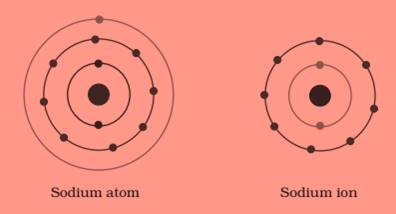Since the atomic number of sodium atom is 11, it has 11 electrons. A positively charged sodium ion (Na+) is formed by the removal of one electron from a sodium atom. So, a sodium ion has 11–1 = 10 electrons in it. Thus, electronic distribution of sodium ion will be 2, 8. The atomic number of an element is equal to the number of protons in its atom. Since, sodium atom and sodium ion contain the same number of protons, therefore, the atomic number of both is 11.

Question 43

In the Gold foil experiment of Geiger and Marsden, that paved the way for Rutherford’s model of an atom, ~ 1.00% of the α-particles were found to deflect at angles > 50o. If one mole of α-particles were bombarded on the gold foil, compute the number of α-particles that would deflect at angles less than 500.

Percent of α-particles deflected more than 500 =1% of α-particles.

Percent of α-particles deflected less than 500 =100–1 = 99%

Number of α-particles bombarded = 1 mole = 6.022×1023 particles

Number of particles that deflected at an angle less than 500

=(99/ 100) × 6.022 ×1023

= (596.178 / 100) x10 23

=5.96x 1023

70%